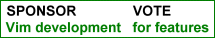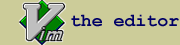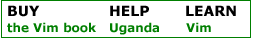mathematic.vim : for inputting mathematic symbols.

 created by Rykka f script type utility description This is a vim keymap file contains mathematic symbols. with some effort to reduce typing conflict and increase typing speed. Also contains a keyhelper for help inputting. - Superscript and Subscript :         `0 ⁰  `+ ⁺ ... ( 0-9 + - ( ) / ^ . , > < )         `a ᵃ  `A ᴬ ... ( a-z except q , Some of A-Z)         _0 ₀  _+ ₊ ... ( 0-9 + - ( ) / ^ )         _a ₐ  _e ₑ ... (a e h i k l m n o p r s t u v x) - Short CMD  :         Greek Alphabet:                     \GA  => Α , \GD   => Δ                     \Ga  => α , \Gg   => γ         Fractions   :                     \1/2   => ½ , \1/3 => ⅓                     \2/3   => ⅔ , \3/5 => ⅗         Arrows      :                     \<--   => ←  , \--v => ↓  , \==>  => ⇒                     \<---  => ⟵  , \--^ => ↑  , \===> => ⟹         Grouping    :                     \(U    => ⎛  , \]U  => ⎤                     \(M    => ⎜  , \]M  => ⎥                     \(B    => ⎝  , \]B  => ⎦                     \(V    => ︵ , \]V  => ︺         Operator    :                     \U.    => ⨃ , \UU   => ∪  , \UN  => ∩                     \I1    => ∫ , \I3   => ∭  , \I=  => ⨎                     \IO1   => ∮ , \IO3  => ∰  , \IOW => ∳                     \PDF   => ∂ , \THF  => ∴  , \BCS => ∵                     \MUL   => × , \DIV  => ÷                     \SQRT2 => √ , \SQRT3 => ∛ , \SQRT4 => ∜         Relations   :                     \<=    => ≤ , \>=  => ≥   , \!=  => ≠                     \<~    => ⪝ , \>~  => ⪞   , \!>  => ≯                     \o+    => ⊕ , \o-  => ⊖   , \ox  => ⊗                     \O+    => ⨀ , \O+  => ⨁   , \OX  => ⨂         Sets        :                     \SUBO  => ⊂ , \SUBE => ⊆  , \SUBN => ⊄                     \SUPO  => ⊃ , \SUPE => ⊇  , \SUPX => ⊉                     \AND   => ∧ , \OR   => ∨  , \NOT  => ¬                     \XOR   => ⊕                     \EXTS  => ∃ , \EXTN => ∄  , \FALL => ∀                     \BOT   => ⊥ , \TOP => ⊤   , \LFT  => ⊣                     \RGT   => ⊢         Misc        :                     \INF  => ∞  , \PRT  => ∝                     \ARC   => ⌒ , \ANG  => ∠                     \DEGC  => ℃ , \DEGF => ℉                     \OHM   => Ω , \MHO  => ℧  , \ANGS => Å                     \a/c   => ℀ , \a/s  => ℁  , \No   => №                     \TM    =>  , \CO   => ©  , \RO   => ®                     \AE    => Æ , \ae   => æ  , \fi   => ﬁ         Fonts       :                     \BBA   => 𝔸 , \BBH => ℍ   , \BB0  => 𝟘                     \FKH   => ℋ , \FKI => ℑ   , \FKL  => ℒ - Latex CMD:         Based on Vim-Latex suite.         Only contains latex command which have corresponding unicode chars.         Fixed some incorrect mapping of Vim-Latex suite:         (\bigodot \bigoplus \bigotimes \long...arrow \iint ...)         e.g:             \neq     => ≠  ,  \Leftarrow =>  ⇐             \simeq   => ≃  ,  \Re        =>  ℜ             \mathbbA => 𝔸  ,  \mathfrakA =>  𝔄             ...         You can use Vim-Latex suite to check these command.         or this pdf of math symbols.         http://amath.colorado.edu/documentation/LaTeX/Symbols.pdf - Key Helper(`:KeyHelper`):         KeyHelper makes viewing and inputting these symbols easier.         It will dynamicly showing matching keymap with your input.         And press '' in the KeyHelper window to append to file.         And you can input more unicode symbols:         0x2500-0x2800,  0x1D100 - 0x1D1DD install details Extract to your ~/.vim folder. - You can make a mapping to use it easyier:            " use it         map km :set keymap=mathematic         " stop use it         map kn :set keymap=         " view it's details, modify it at your convenience.         map kvm :sp ~/.vim/keymap/mathematic.vim              " show inputting cmd:         " set showcmd         " when a longer time for inputting is needed:         " set timeoutlen=3000         " or disable inputting timeout:         " set notimeout ':h keymap' for further details. - Using KeyHelper: (`:KeyHelper (\)`)         " if your mathematic.vim is not in '~/.vim/bundle/' or '~/.vim/keymap'         " then you should set g:mathematic_user_dir         " let g:mathematic_user_dir = "~/.vim/myplug/keymap/mathematic.vim"         " map KeyHelper with another mapping         " nmap kk :KeyHelper         " if you want fuzzy matching         " let g:mathematic_fuzzymatch = 1 Post issues and suggestions at https://github.com/Rykka/mathematic.vim. To request adding keymap CMDS , please post CMD and Character.

 rate this script Life Changing Helpful UnfulfillingIf you have questions or remarks about this site, visit the vimonline development pages. Please use this site responsibly. Questions about Vim should go to the maillist. Help Bram help Uganda. OSDN